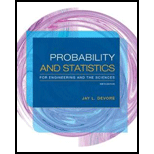# A system consists of two identical pumps, #1 and #2. If one pump fails, the system will still operate. However, because of the added strain, the remaining pump is now more likely to fail than was originally the case. That is, r 5 P (#2 fails | #1 fails). P (#2 fails) = q. If at least one pump fails by the end of the pump design life in 7% of all systems and both pumps fail during that period in only 1%, what is the probability that pump #1 will fail during the pump design life?### Probability and Statistics for Eng...

9th Edition
Jay L. Devore
Publisher: Cengage Learning
ISBN: 9781305251809### Probability and Statistics for Eng...

9th Edition
Jay L. Devore
Publisher: Cengage Learning
ISBN: 9781305251809

#### Solutions

Chapter
Section
Chapter 2.4, Problem 52E
Textbook Problem

## A system consists of two identical pumps, #1 and #2. If one pump fails, the system will still operate. However, because of the added strain, the remaining pump is now more likely to fail than was originally the case. That is, r 5 P(#2 fails | #1 fails). P(#2 fails) = q. If at least one pump fails by the end of the pump design life in 7% of all systems and both pumps fail during that period in only 1%, what is the probability that pump #1 will fail during the pump design life?

Expert Solution
To determine

Find the probability that the pump #1 fails during pump design life.

### Explanation of Solution

Given info:

The system has two identical pumps #1 and #2. The system will operate even if one pump fails. The pumps fail due to the strain that is, r=P(#2 fails | #1 fails)>P(#2 fails)=q. There is 7% chance that at least one pump fails at the end of pump design life and 1% chance that both pumps fail at the end.

Calculation:

Here A1 denotes the event that pump #1 fails, A2 denotes the event that pump #2 fails,

A1A2 denotes failure of at least one pumps, and A1A2 denotes failure of both pumps. Also, P(A1A2)=0.07 and P(A1A2)=0.07.

Since the two pumps were identical, so P(A1)=P(A2)

For any two events A and B,

P(A

### Want to see the full answer?

Check out a sample textbook solution.See solution

### Want to see this answer and more?

Bartleby provides explanations to thousands of textbook problems written by our experts, many with advanced degrees!

See solution